# 日拱一卒，伯克利教你Python核心技術，迭代器和生成器# Topics

## Iterables and Iterators

```python for item in iteralbe: # do something```

for迴圈可以使用在任何可迭代物件（iterable）中。我們之前描述for迴圈的時候，說的是它可以用在任何序列上——所有的序列都是可迭代的。但除了序列之外也有其他物件也是可迭代的。實際上，for迴圈會被直譯器轉錄成類似下面這樣的程式碼：

```python iterator = iter(iterable) try: while True: elem = next(iterator) # do something except StopIteration: pass```

• 首先，`iter`是一個內建函式，它應用在可迭代物件上，生成一個對應的迭代器。迭代器是一個可以在可迭代物件上迭代的物件，它會一直記錄下一個被迭代的元素
• `next`函式應用在迭代器上，用來獲取序列中的下一個元素
• 當序列中沒有下一個元素時，會丟擲`StopIteration`異常。在for迴圈當中，這個異常會被捕獲，程式可以繼續執行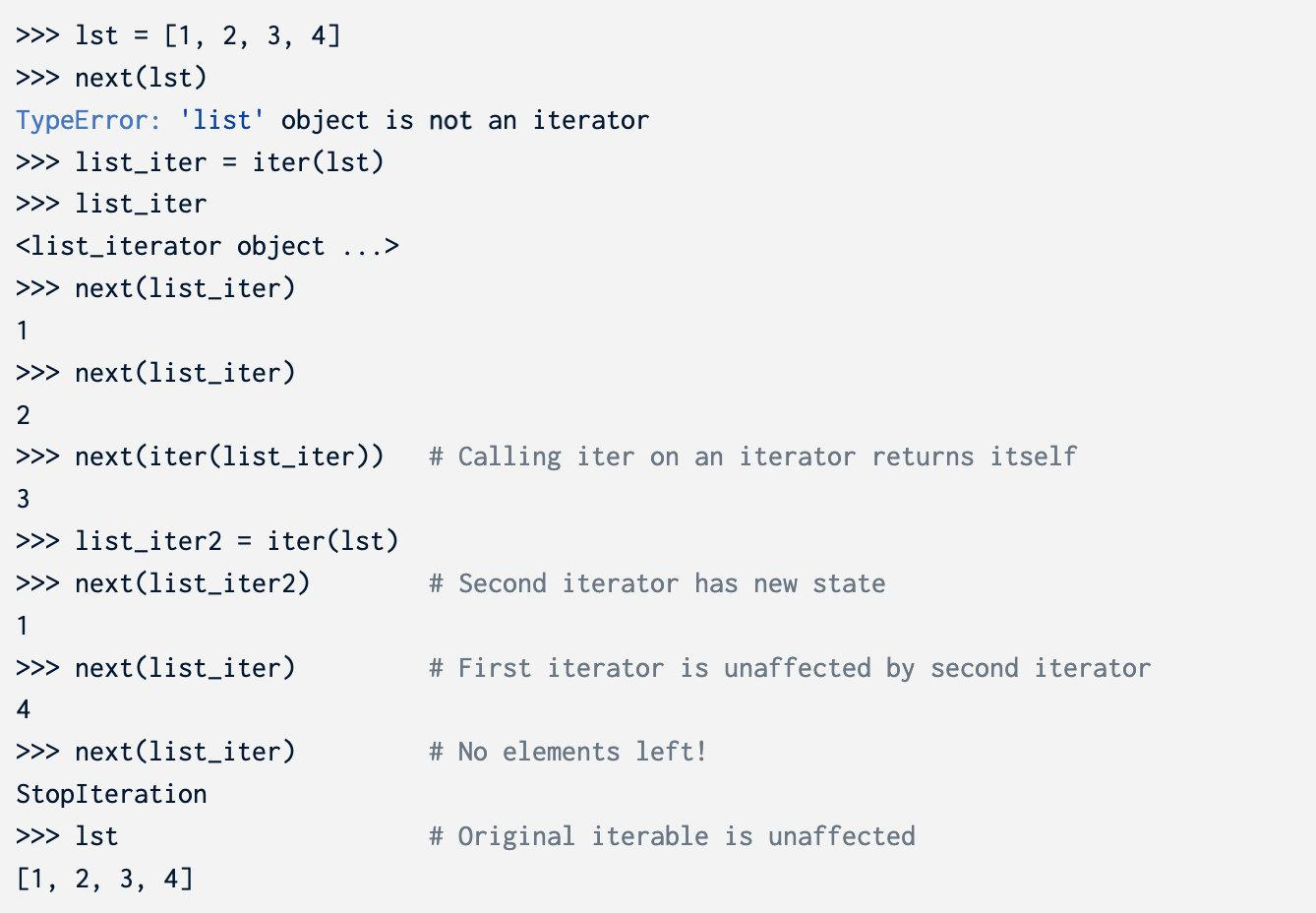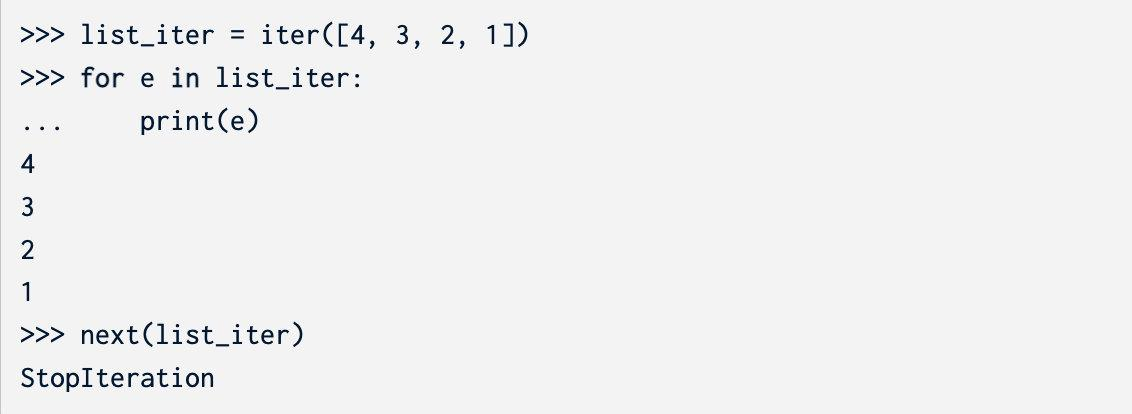### Iterable uses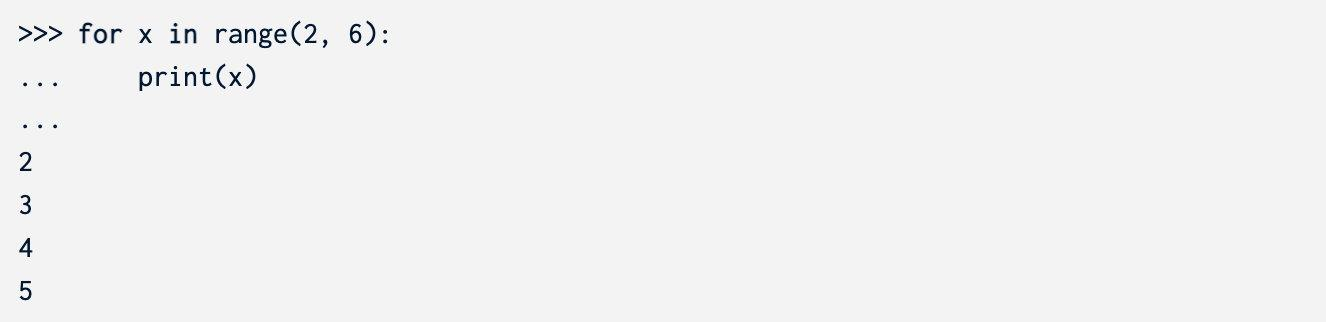• map(f, iterable) - 建立一個迭代器，對iterable中的x，得到`f(x)`
• filter(f, iterable) - 建立一個迭代器，對iterable中的x，得到所有`f(x) == True`的x
• zip(iter1, iter2) - 對`iter1`中的所有x和`iter2`中的所有y，建立一個迭代器，得到所有的`(x, y)`的pair
• reversed(iterable) - 建立一個迭代器可以反向遍歷`iterable`
• list(iterable) - 建立一個list，得到`iterable`中的所有元素
• tuple(iterable) - 建立一個tuple，包含`iterable`中的所有元素
• sorted(iterable) - 建立一個排好序的list，包含`iterable`中的所有元素

## Generators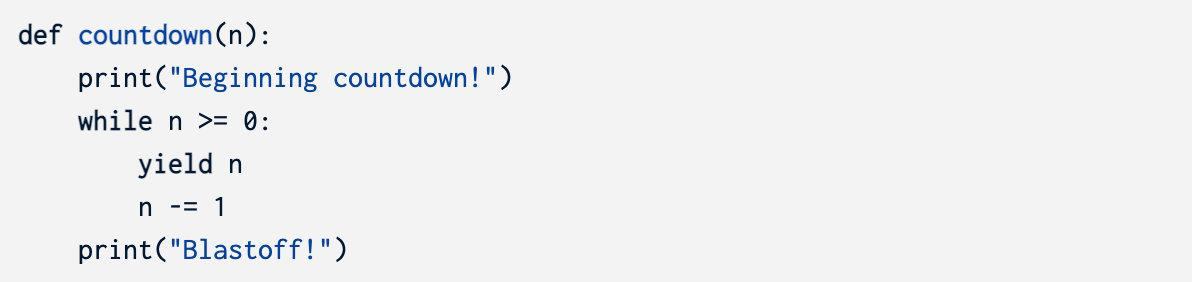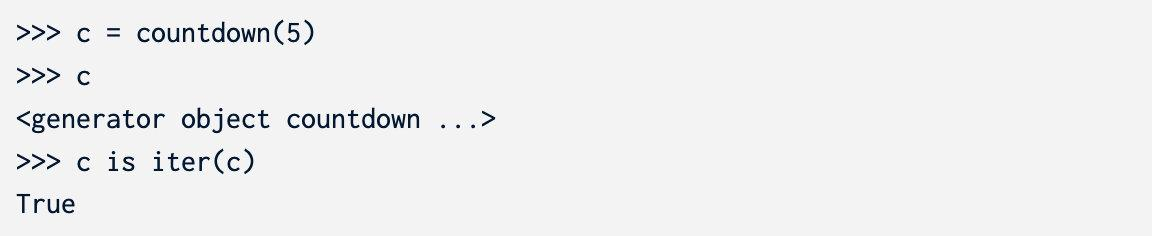`next`被呼叫的時候，程式會從函式主體的第一行開始執行，一直到遇到`yield`語句。`yield`語句之後的內容將被evaluate並返回。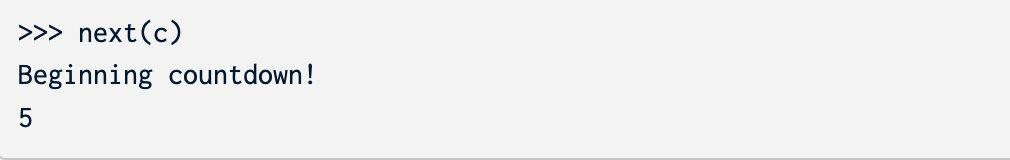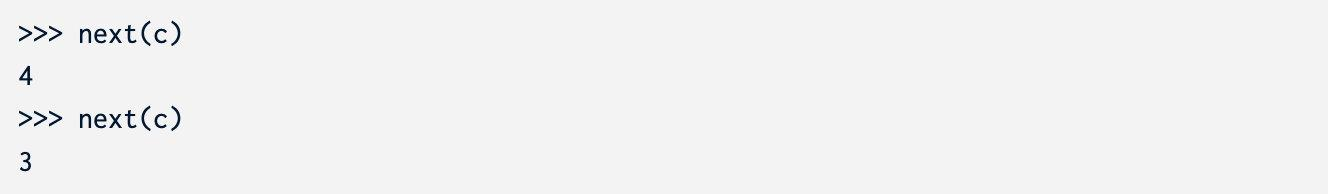`countdown`多次呼叫會建立不同的生成器，它們擁有專屬的狀態。通常，生成器不會重啟。如果你想要重新得到一個序列，你可以建立另外一個生成器物件。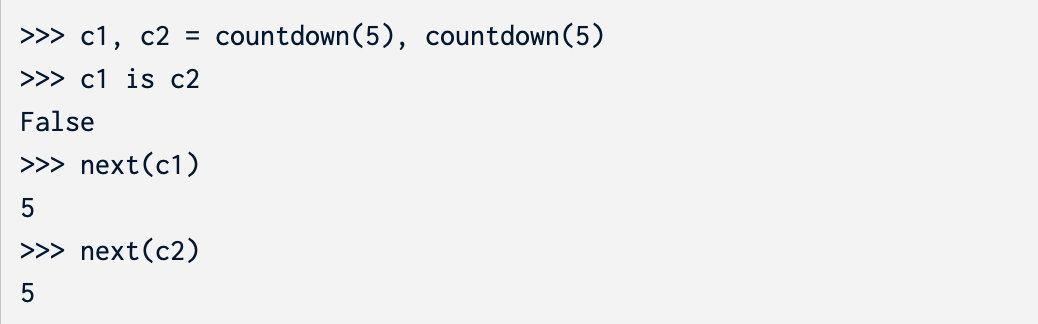• 生成器函式擁有`yield`語句，並且會返回一個生成器物件

• 對生成器物件呼叫`iter`會得到一個同樣的物件，並且不會修改生成器的狀態

• 生成器的函式主體不會被執行，直到呼叫`next`函式。對一個生成器物件呼叫`next`函式，會執行並且返回序列中的下一個元素。如果序列已經結束了，丟擲`StopIteration`異常
• 生成器會記住下一次執行`next`時的狀態。

• 第一次呼叫`next`時：

• 進入函式，執行程式知道遇到`yield`
• 返回`yield`語句中的值，記住`yield`語句的位置
• 持續呼叫`next`時：

• 從上一次`yield`語句的下一行開始執行，遇見`yield`停止
• 返回`yield`語句中的值，記住當前執行的位置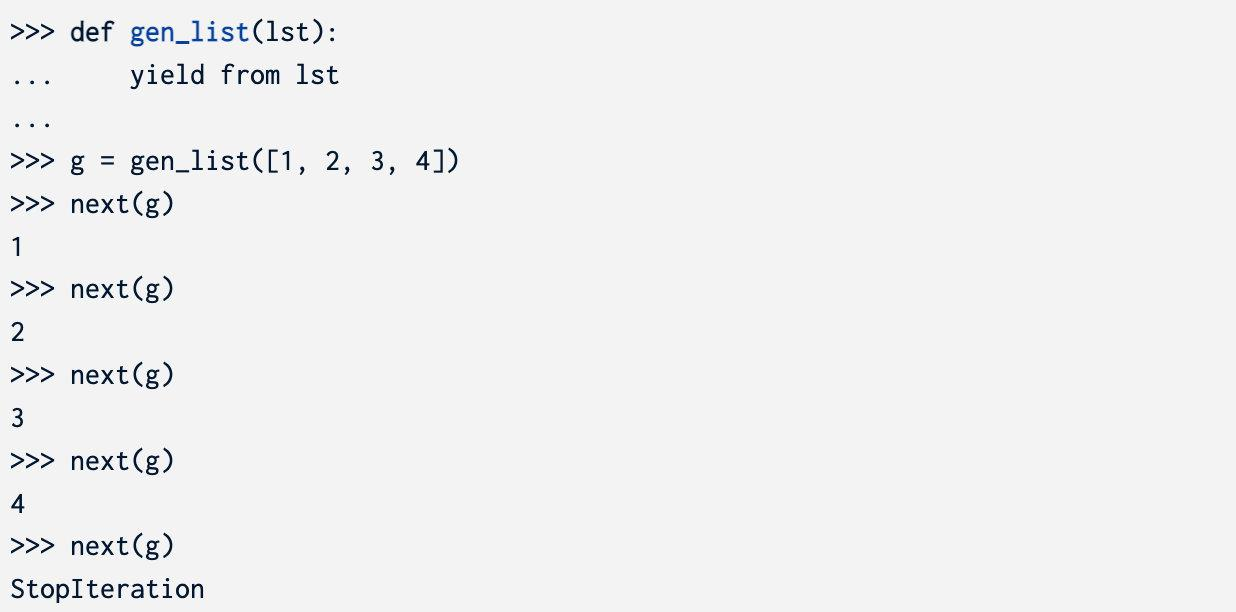# Required Questions

## WWPD

### Q1: WWPD: Iterators

```python

s = [1, 2, 3, 4] t = iter(s) next(s)

next(t)

next(t)

iter(s)

next(iter(s))

next(iter(t))

next(iter(s))

next(iter(t))

next(t)

r = range(6) r_iter = iter(r) next(r_iter)

[x + 1 for x in r]

[x + 1 for x in r_iter]

next(r_iter)

list(range(-2, 4)) # Converts an iterable into a list

map_iter = map(lambda x : x + 10, range(5)) next(map_iter)

next(map_iter)

list(map_iter)

for e in filter(lambda x : x % 2 == 0, range(1000, 1008)): ... print(e) ...

[x + y for x, y in zip([1, 2, 3], [4, 5, 6])]

for e in zip([10, 9, 8], range(3)): ... print(tuple(map(lambda x: x + 2, e))) ...

```

### Q2: WWPD: Generators

```python def gen(): print("Starting here") i = 0 while i < 6: print("Before yield") yield i print("After yield") i += 1

next(gen)

gen

g = gen() g

g == iter(g)

next(g)

next(g)

next(g)

g2 = gen() next(g2)

iter(g2)

next(iter(g))

next(gen())

```

## Coding Practice

### Q3: Scale

```python def scale(s, k): """Yield elements of the iterable s scaled by a number k.

``````>>> s = scale([1, 5, 2], 5)
>>> type(s)
<class 'generator'>
>>> list(s)
[5, 25, 10]

>>> m = scale(naturals(), 2)
>>> [next(m) for _ in range(5)]
[2, 4, 6, 8, 10]
"""
``````

```

#### 答案

```python def scale(s, k): for i in s: yield i * k```

```python def scale(s, k): yield from map(lambda x: x * k, s)```

### Q4: Trap

```python def trap(s, k): """Return a generator that yields the first K values in iterable S, but raises a ValueError exception if any more values are requested.

``````>>> t = trap([3, 2, 1], 2)
>>> next(t)
3
>>> next(t)
2
>>> next(t)
ValueError
>>> list(trap(range(5), 5))
ValueError
>>> t2 = trap(map(abs, reversed(range(-6, -4))), 2)
>>> next(t2)
5
>>> next(t2)
6
>>> next(t2)
ValueError
"""
``````

```

#### 答案

```python def trap(s, k): it = iter(s) for _ in range(k): yield next(it) raise ValueError("no more values")```

# Optional Questions

### Q5: Hailstone

1. 選擇一個正整數`n`作為開始
2. 如果`n`是偶數，將它除以2
3. 如果`n`是奇數，將它乘3再加1
4. 重複以上過程，直到`n`等於1

```python def hailstone(n): """ >>> for num in hailstone(10): ... print(num) ... 10 5 16 8 4 2 1 """ "*** YOUR CODE HERE ***"```

#### 答案

```python def hailstone(n): while n != 1: yield n if n % 2 == 0: n //= 2 else: n = n * 3 + 1 yield n```

### Q6: Repeated

```python def repeated(t, k): """Return the first value in iterable T that appears K times in a row.

``````>>> s = [3, 2, 1, 2, 1, 4, 4, 5, 5, 5]
>>> repeated(trap(s, 7), 2)
4
>>> repeated(trap(s, 10), 3)
5
>>> print(repeated([4, None, None, None], 3))
None
"""
assert k > 1
``````

```

#### 答案

```python def repeated(t, k): assert k > 1 "*** YOUR CODE HERE ***" it = iter(t) last, cnt = next(it), 1 for i in it: if i == last: cnt += 1 if cnt == k: return i else: last, cnt = i, 1```

### Q7: Merge

```python def merge(s0, s1): """Yield the elements of strictly increasing iterables s0 and s1, removing repeats. Assume that s0 and s1 have no repeats. s0 or s1 may be infinite sequences.

``````>>> m = merge([0, 2, 4, 6, 8, 10, 12, 14], [0, 3, 6, 9, 12, 15])
>>> type(m)
<class 'generator'>
>>> list(m)
[0, 2, 3, 4, 6, 8, 9, 10, 12, 14, 15]
>>> def big(n):
...    k = 0
...    while True: yield k; k += n
>>> m = merge(big(2), big(3))
>>> [next(m) for _ in range(11)]
[0, 2, 3, 4, 6, 8, 9, 10, 12, 14, 15]
"""
i0, i1 = iter(s0), iter(s1)
e0, e1 = next(i0, None), next(i1, None)
``````

```

#### 答案

```python def merge(s0, s1): i0, i1 = iter(s0), iter(s1) e0, e1 = next(i0, None), next(i1, None) "*** YOUR CODE HERE ***" while e0 is not None or e1 is not None: if e0 is None: yield e1 e1 = next(i1, None) elif e1 is None: yield e0 e0 = next(i0, None) else: if e0 == e1: yield e0 e0, e1 = next(i0, None), next(i1, None) elif e0 < e1: yield e0 e0 = next(i0, None) else: yield e1 e1 = next(i1, None)```

### Q8: Remainder Generator

`remainders_generator`讀入一個整數`m`，yield `m`個不同的生成器。第一個生成器是`m`的倍數（對m取模餘數為0），第二個生成器是對`m`取模餘數為1的生成器，以此類推。

```python def remainders_generator(m): """ Takes in an integer m, and yields m different remainder groups of m.

``````>>> remainders_mod_four = remainders_generator(4)
>>> for rem_group in remainders_mod_four:
...     for _ in range(3):
...         print(next(rem_group))
0
4
8
1
5
9
2
6
10
3
7
11
"""
``````

```

#### 答案

```python def remainders_generator(m): def inner(r): while True: yield r r += m

``````for i in range(m):
yield inner(i)
``````

```

### Q9: Zip Generator

```python def zip_generator(*iterables): """ Takes in any number of iterables and zips them together. Returns a generator that outputs a series of lists, each containing the nth items of each iterable. >>> z = zip_generator([1, 2, 3], [4, 5, 6], [7, 8]) >>> for i in z: ... print(i) ... [1, 4, 7] [2, 5, 8] """ "*** YOUR CODE HERE ***"```

#### 答案

```python def zip_generator(*iterables): itors = [iter(it) for it in iterables] while True: yield [next(it) for it in itors]```